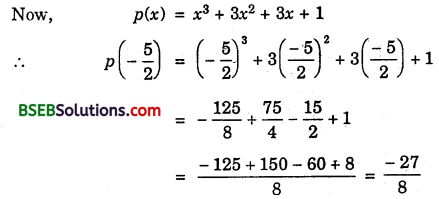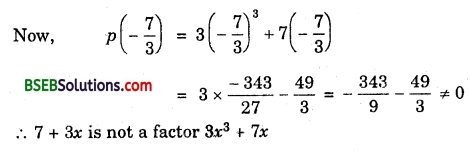# Bihar Board Class 9th Maths Solutions Chapter 2 Polynomials Ex 2.3

Bihar Board Class 9th Maths Solutions Chapter 2 Polynomials Ex 2.3 Textbook Questions and Answers.

## BSEB Bihar Board Class 9th Maths Solutions Chapter 2 Polynomials Ex 2.3Question 1.
Find the remainder when x³ + 3x² + 3x + 1 is divided by
(i) x + 1
(ii) x – $$\frac { 1 }{ 2 }$$
(iii) x
(iv) x + π
(v) 5 + 2x
Solution:
(i) By remainder theorem, the required remainder is equal to p(- 1).
Now, p(x) = x³ + 3x² + 3x + 1
∴ p(- 1) = (- 1)³ + 3(- 1)² + 3(- 1) + 1
= – 1 + 3 – 3 + 1 = 0
Hence, required remainder = p(- 1) = 0

(ii) By remainder theorem, the required remainder is equal p($$\frac { 1 }{ 2 }$$)(iii) By remainder theorem, the required remainder is equal to p(0).
Now, p(x) = x³ + 3x² + 3x + 1
∴ p(0) = 0 + 0+ 0 + 1 = 1
Hence, the required remainder = p(0) = 1

(iv) By remainder theorem the required remainder is p(~ n)
Now p(x) = x³ + 3x² + 3x + 1
∴ p(- π) = (- π)³ + 3(- π)² + 3(- π) + 1
= – π³ + 3π² – 3π + 1

(v) By remainder theorem, the required remainder is 5 p(- $$\frac { 5 }{ 2 }$$)Question 2.
Find the remainder when x³ – ax² + 6x – a is divided by x – a.
Solution:
Let p(x) = x³ – ax² + 6x – a
By remainder theorem, when p(x) is divided by x – a. Then, remainder = p(a)
∴ p(x) = a³ – a . a² + 6a – a
= a³ – a³ + 6a – a = 5aQuestion 3.
Check whether 7 + 3x is a factor of 3x² + 7x
Solution:
7 + 3x will be a factor of p{x) = 3x² + 7x if p(-$$\frac { 7 }{ 3 }$$) = 0